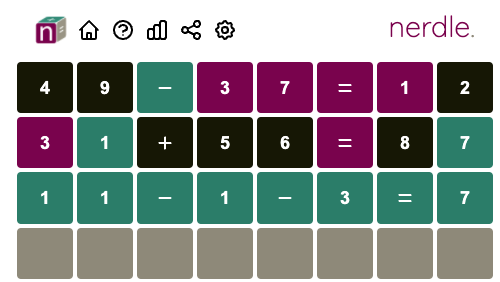# From Wordle to Nerdle

About two months ago I wrote a blogpost about what strategies you could use to solve Wordle in the most efficient way. In the end I wrote a program that looks ahead a single guess and finds the word that gives you the most information.

The main hype around Wordle (and all of the clones), seems to have settled down now… but I still play some of them.

## Nerdle

One clone I really enjoyed playing the last couple of weeks has been Nerdle. In the game Nerdle all your guesses need to be a valid mathematical calculation. For example: `31 + 56 = 87`.In this game, you can’t just write down anything, each and every try needs to be a valid calculation.

### Generating the guesses

Yesterday, when I had a bit of time with a colleague, we set about trying to adapt the Wordle-bot into a Nerdle-bot. In theory this would be extremely easy, the only hard part is getting a valid “wordlist”.

When I created the Wordle bot, I just downloaded a list of all valid Wordle words. But in this case I set myself the challenge to create all the valid guesses from scratch.

### Patterns

First, let’s look at some of the rules. There are 8 characters you can fill, and the final part will always be:

• `=N` with N is a single digit number between 0-9
• `=NN` with NN being between 10 and 99; or
• `=NNN` with NNN being between 100 and 999

It’s easy to generate all those options. However: the part before that is a little bit harder.

Calculations can, because of the limited size, be just one of two patterns (of various sizes): `Value1 Operator Value2` or `Value1 Operator Value2 Operator Value3`

For example `12+34=46` or `10/2+1=6`

These patterns are build up from left to right, starting first with a single digit number, appending the first operator, etc, until we have all valid 4, 5 or 6-character calculations.

Now obviously, the tricky part is that we need to evaluate all these calculations and check if the result fits in the amount of characters we have left, for example `=NN`! This code isn’t pretty… at all, even though I tried to make it generic. I won’t share it haha.

### Bugs/problems

I quickly ran into the first big obstacle: How do you calculate the result of something like `1+10/2`? You’ll first need to evaluate `10/2`, store the result, and finally do `1+5` and check if `=6` fits (which it does).

After writing some code and making sure we always evaluate in the correct order in case of `Value1 Operator Value2 Operator Value3` I thought we were done. It showed me there are about 13k valid guesses. And this was wrong.

The first bug I encountered was silly. I falsely put in a rule that no result can be zero. But in some cases zero’s are allowed, for example ending with `=0`. After fixing this bug I found out I was still missing some valid guesses.

After diff-ing my list with a list I found online I noticed I was missing things like: `9/6*8=12`

Because Nerdle doesn’t handle fractions, I thought that the divides should never have remainders. Thus I marked `9/6` invalid because it doesn’t evenly divide. However… we are multiplying afterwards (!) so the actual result (12) IS a valid guess for Nerdle.

### Results

After fixing all the bugs I had a complete list of all valid Nerdle guesses, and here are some stats:

There are 17723 valid Nerdle answers

• 6839 of which contain a `+`
• 7810 of which contain a `-`
• 5574 of which contain a `*`
• 4024 of which contain a `/`
• 8607 of which contain two math operators
• 5890 end with `=N`
• 10515 end with `=NN`
• 1318 of which end with `=NNN`

And when I fired up the Wordle-checker, my code thinks the best start is: `49-37=12`

Other great starters are: `39-24=15` `34+27=61` `9+8/2=13` `7+8-13=2`

After writing the bot I’ve tried it against the last three Nerdle challenges, and each game was solved after just three guesses!

For example yesterday the bot calculated the following guesses:

``````49-37=12 : ⬛⬛🟩🟪🟪🟪🟪⬛
31+56=87 : 🟪🟩⬛⬛⬛🟪⬛🟩
``````

After just these two guesses there is only ONE valid guess left: `11-1-3=7`: 🟩🟩🟩🟩🟩🟩🟩🟩